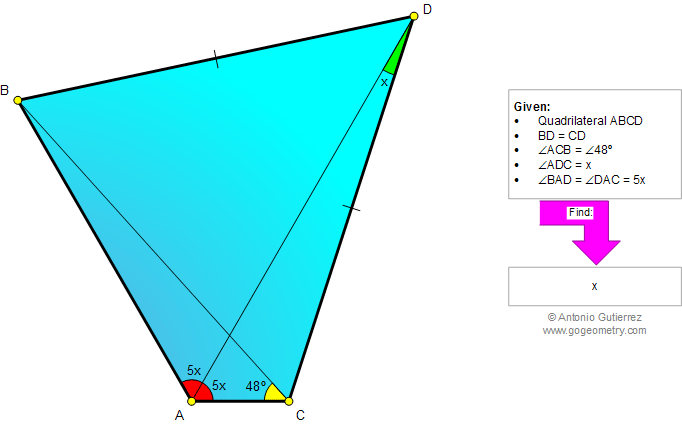###### Online Geometry Problem 873: Quadrilateral, Diagonal, Triangle, Angle Bisector, Congruence. Level: High School, College, Mathematics Education
 The figure below shows a quadrilateral ABCD with BD = CD, angle ACB = 48 degrees, angle ADC = x, and angle BAD = angle = DAC = 5x. Find x.Home | Search | Geometry | Problems | All Problems | Open Problems | Visual Index | 10 Problems | 871-880 | Triangles | Quadrilateral | Angles | Email | Solution / comment | By Antonio Gutierrez